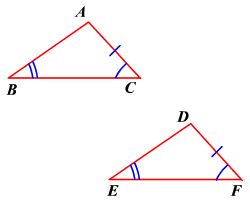# AAS (Angle-Angle-Side) Postulate

If two angles and a non-included side of a triangle are congruent to two angles and the corresponding non-included side of another triangle, then the two triangles are congruent .In the figure, .

Therefore, by the AAS postulate, $\Delta ABC\cong \Delta DEF$ .